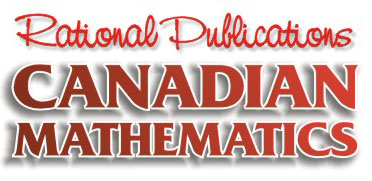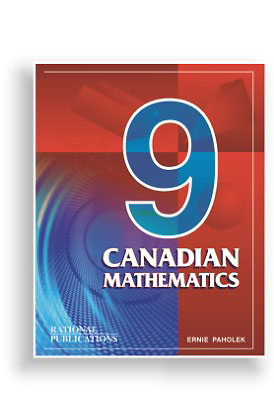The following summary contains a complete listing of the chapters, along with a sample question from each chapter.

Should you have further questions about the text book, please contact us.

If you wish to order Canadian Mathematics 9 or any of the other Rational Publications text books, please do so by clicking on the order form.

 CHAPTER 1 - RATIONAL NUMBERS 1.1 Integers 1.2 Decimals 1.3 Fractions 1.4 Order of Operations 1.5 Fractions <—> Decimals <—> Percent 1.6 Percent Conditions 1.7 Properties of Whole Numbers 1.8 Review CHAPTER 2 - EQUATIONS & INEQUALITIES 2.1 Solving Type I Equations 2.2 Solving Type II Equations 2.3 Solving Type III Equations 2.4 Solving Type IV Equations 2.5 Solving Ratio Equations 2.6 Inequalities & Graphing 2.7 Equations with Letters 2.8 Review CHAPTER 3 - RATIO & PROPORTION AND FORMULA PROBLEMS 3.1 Rate & Ratio Problems 3.2 General Formula Problems 3.3 Rate of Work Problems 3.4 Percent Problems 3.5 Distance Problems 3.6 Cost Problems 3.7 Discount Problems 3.8 Average Problems 3.9 The Selling Formula 3.10 Commission Problems 3.11 Interest Problems 3.12 Sale, GST & Basic Income Tax 3.13 Property Tax 3.14 Review CHAPTER 4 - ALGEBRAIC PROBLEMS 4.1 Translation (Math <—> English) 4.2 Number Problems 4.3 Geometric Problems 4.4 Old-Fashioned Problems 4.5 Age Problems 4.6 Coin Problems 4.7 Mixture Problems 4.8 Distance (dA + dB = dT) 4.9 Distance (dA = dB) - 51 4.10 Review CHAPTER 5 - GEOMETRY 5.1 Angles in Mathematics 5.2 Interior Angle Sum 5.3 Geometric Constructions     1. Congruent Segments     2. Congruent Angles     3. Perpendicular Bisector     4. Perpendicular (Point Not On Line)     5. Perpendicular (Point On a Line)     6. Angle Bisector     7. Parallel Lines (Rhombus Method)     8. Parallel Lines (Corresponding ?'s)     9. Equilateral Triangles     10. Isosceles Triangles     11. Angles     12. Congruent Triangles (SSS)     13. Congruent Triangles (SAS)     14. Congruent Triangles (ASA)     15. Inscribed Square     16. Rhombus     17. Rectangle     18. Parallelogram 5.4 Review CHAPTER 6 - MEASUREMENT 6.1 Metric Conversion 6.2 Volume, Capacity & Mass 6.3 Perimeter of Polygons 6.4 Area of Polygons 6.5 Review 6.6 Area of Shaded Regions CHAPTER 7 - PYTHAGORAS 7.1 Labelling Vertices & Sides 7.2 Labelling Right Angle Triangles 7.3 Area of Squares 7.4 Triplets & Triads 7.5 Squares Roots 7.6 Calculations CHAPTER 8 - TRIGONOMETRY 8.1 Right Angle Triangle Calculations 8.2 Trigonometry Tables CHAPTER 9 - PRISMS, PYRAMIDS, CONES & SPHERES 9.1 Definitions 9.2 Rectangular Prisms 9.3 Square Prisms 9.4 Cubes 9.5 Triangular Prisms 9.6 Regular Polygonal Prisms 9.7 Cylinders 9.8 Review 9.9 Volume of Prisms 9.10 Volume of Pyramids & Cones 9.11 Area & Volume of Spheres 9.12 Review (Area & Volume) CHAPTER 10 - EXPONENTS 10.1 Positive & Negative Exponents 10.2 Multiplication with Exponents 10.3 Division with Exponents 10.4 Power of a Power Property 10.5 Calculations with Exponents 10.6 Scientific Notation 10.7 Multiplication & Division 10.8 Square Roots & Cube Roots 10.9 Radicals (x and ?÷) 10.10 Radicals (+ and –) 10.11 Review CHAPTER 11 - ALGEBRA 11.1 Geometric Definitions 11.2 Substitution & Calculation 11.3 Monomials (+ and –) 11.4 Polynomials (+ and –) 11.5 Algebra Tiles (+ and –) 11.6 Monomials (x and ÷) 11.7 Polynomials (x and ÷) 11.8 Division of Polynomials 11.9 Review 11.10 Factoring     1. Common Factoring     2. Factoring by Grouping     3. Difference of Squares     4. Trinomials (No coefficient)     5. Trinomials (Coefficients) 11.11 Reducing Rational Expressions 11.12 Rational Expressions (x and ÷) 11.13 Rational Expressions (+ and –) 11.14 Rational Number Review CHAPTER 12 - THE COORDINATE PLANE 12.1 Plotting Points 12.2 Transalations (Slides) 12.3 Reflections (Flips) 12.4 Rotations (Turns) 12.5 Dilations 12.6 Linear Graphs 12.7 Parabolas 12.8 Hyperbolas 12.9 Review CHAPTER 13 - DATA MANAGEMENT 13.1 Statistics 13.2 Characteristics of Data 13.3 Box and Whisker Plots 13.4 Stem and Leaf Plots 13.5 Displaying Data 1. Broken Line Graphs     2. Bar Graphs     3. Circle Graphs 13.6 Probability 13.7 Probability (Independent Events) CHAPTER 14 - CIRCLE GEOMETRY 14.1 Angles Within a Circle 14.2 Chord Properties 14.3 Tangents 14.4 Circle Geometry ReviewHome   |   Math 7   |   Math 8   |   Order Form   |   Contact Us